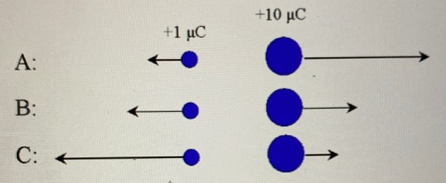# Problem: Two charges q = + 1 μc and Q = +10 μC are placed near each other as shown in the figure. 1) Which of the following diagrams best depicts the forces acting on the charges: ○ A○ B○ C 2) Briefly explain your answer to the previous question.

###### FREE Expert Solution

1)

Coulomb's law:

$\overline{){\mathbf{F}}{\mathbf{=}}\frac{\mathbf{k}{\mathbf{q}}_{\mathbf{1}}{\mathbf{q}}_{\mathbf{2}}}{{\mathbf{r}}^{\mathbf{2}}}}$, where k is Coulomb's constant and r is the distance between the charges q1 and q2.

87% (306 ratings)###### Problem Details

Two charges q = + 1 μc and Q = +10 μC are placed near each other as shown in the figure.1) Which of the following diagrams best depicts the forces acting on the charges:

○ A
○ B
○ C